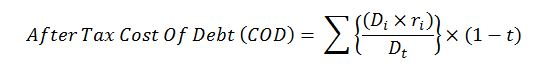## Cost of DebtCost of Debt (COD) is an effective rate that a company will pay on it current debt. The Cost of Debt (COD) can be measured in term before or after tax COD however the after tax Cost of Debt (COD) is often used.

After tax Cost of Debt (COD) is mathematically expressed in the equation below;Where;

Di = individual loan amounts

ri = interest rate of the individual loan

Dt = total loans that a company has

t = company corporate tax rate, %

Note: the interest payment on loans is classified as an operating expense and it is a tax deductible. Continue reading